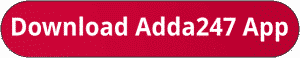Latest Banking jobs   »   RBI Grade B Phase 1 quant

# Quantitative Aptitude Quiz For RBI Grade B Phase 1 2023 – 06th July

Directions (1-5): Pie chart given below shows percentage distribution of male population of five villages. Study the pie chart carefully and answer the following questions.Note: Ratio between the total male population to total female population is 10 : 7.Q3. Find the difference between the average female population of village B and C together and the average female population of D and E together if percentage distribution of male and female in each village is same?
(a) 150
(b) 200
(c) 175
(d) 300
(e) 250

Q4. Ratio of female population of village C to that of village D is 2 : 3 and the ratio of female population of village D to male population of village E is 4 : 5 then find the total female population of village D and C together ?
(a) 300
(b) 750
(c) 120
(d) 450
(e) 600Directions (6 – 10): Given below the table shows Investment of five person, time and share of profit. Some data are missing, calculate that according to the question and answer the questions –Q6. P, Q and S invested in a business together, investment of P is 75% of investment of S and all three invested for same time. If Q and S gets extra 12% and 15% of total profit respectively and remaining profit is distributed according to their share, then find the total profit.
(a) 270000 Rs.
(b) 370000 Rs.
(c) 375000 Rs.
(d) 275000 Rs.
(e) 325000 Rs.

Q7. Person Q and R invested in the ratio of 4 : 3 and ratio between time period of Investment Q to R is 5 : 3. If both person agree that 65% of the total profit should be divided equally and remaining profit is to be divided into ratio of their capital. If Q gets 10010 Rs. more than R. then find the total profit share of R ?
(a) 27765 Rs.
(b) 28190 Rs.
(c) 42497 Rs.
(d) 34307 Rs.
(e) 32695 Rs.

Q8. Q started a business with his investment, after some month S came to joined with him and invest his amount in business. At the end of 3 year, the ratio of profit of Q to S is 3 : 2. Find after how many month S joined the business ?
(a) 4 months
(b) 6 months
(c) 3 months
(d) 5 months
(e) 8 months

Q9. If T received Rs. 24300 as profit out of the total profit of Rs. 40500 which T and R earned at the end of one year. If T invested Rs. 81000 for 9 months, whereas R invested his amount for the whole year, what was the amount invested by R ?
(a) 30500 Rs.
(b) 40500 Rs.
(c) 35500 Rs.
(d) 32500 Rs.
(e) 40050 Rs.

Q10. If Q and R invested into the ratio of 8 : 9 and R and S Invested into the ratio of 3 : 2. At the end of the year if they all got a total profit of 37030 Rs. then find the share of profit of Q, R and S individually ?
(a) 14490 Rs, 9880 Rs, 12880 Rs.
(b) 9660 Rs, 17710 Rs, 11005 Rs.
(c) 12880 Rs, 14490 Rs, 9660 Rs.
(d) 12434 Rs, 13594 Rs, 11006 Rs.
(e) None of these

Solutions:## FAQs

### How many sections are there in the RBI Grade B Phase 1 Exam?

There are 4 sections in the RBI Grade B Phase 1 Exam i.e. English Language, General Awareness, Quantitative Aptitude & Reasoning.